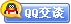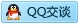##用户名 Email 自动登录 找回密码 密码 会员注册
 VIP会员，3年作业免费下 ！ 奥鹏作业，奥鹏毕业论文检测 新手作业下载教程，充值问题 没有找到答案，请在此处留言！ 2019年12月最新全国统考资料 投诉建议，加盟合作！奥鹏课程积分软件(ver:3.1)

# 东大19秋学期《电路原理》在线平时作业2（参考）发表于 2019-9-7 03:01:26 | 显示全部楼层 |阅读模式【奥鹏】[东北大学]19秋学期《电路原理》在线平时作业2 试卷总分:100    得分:100 第1题,如图理想变压器的电压方程为(     )。A u1=nu2 ,         B u1=-nu2 ,       C 其它 A、A B、B C、C D、D 正确答案: 第2题,图中，L=20mH, C=50pF, R=1kW, ù=10D00 V。求电路的电流I为 (      ) mA 。A I=￥         B I=10       C 其它 A、A B、B C、C D、D 正确答案: 第3题,路图中的时间常数为(      )。A t =103           B t =10-3               C 其它 A、A B、B C、C D、D 正确答案: 第4题,图中，L=10mH，C=10mF，ù=1D00 V。电路的谐振频率f0为(      )赫兹。A 106   其它      B 15915       C A、A B、B C、C D、D 正确答案: 第5题,如图空心变压器的输出的特性方程为(      )。A u2=L1(di2/dt)-M(di1/dt),     B u2=L2(di2/dt)-M(di12/dt),     C 其它 A、A B、B C、C D、D 正确答案: 第6题,滤波器是利用电阻的频率特性实现滤掉某些频率波形的设备。(         ) A、错误 B、正确 正确答案: 第7题,图示电路，t=0 时将开关K闭合，t0时电路已达稳态，电压u2(0)=10V 。(      ) A、错误 B、正确 正确答案: 第8题,星形联接的三相电路可以是三线制也可以是四线制。(         ) A、错误 B、正确 正确答案: 第9题,图中电位Ua=6V。(     ) A、错误 B、正确 正确答案: 第10题,电路如图所示， t0时电路已达稳态，t=0时开关Ｋ由①扳向②，电压uC(0)=4V 。(      ) A、错误 B、正确 正确答案: 第11题,Y11等于双口网络输出端短路时的输入导纳。(        ) A、错误 B、正确 正确答案: 第12题,已知 R=1Ω, C=1F, a=2。 e(t)=e-at1(t)V,时的uC(t)=e-t 1(t)V（      ）。 A、错误 B、正确 正确答案: 第13题,图中 US=10V, R=10Ω, IS=1A。则电流源输出的电功率为20W。(      ) A、错误 B、正确 正确答案: 第14题,图中70V电压源发出的功率P70=420W。(      ) A、错误 B、正确 正确答案: 第15题,对称三相电源线电压为380V，Z=6+j4W， Zl =2+j2W。则负载Z的相电流IP=22A。（       ） A、错误 B、正确 正确答案: 第16题,图中电流I=1.5A。（    ） A、错误 B、正确 正确答案: 第17题,已知 R1=R2=1Ω, L1=1H, C=1F。若激励是 d(t)，响应是u2(t)，网络函数H(S)=1/(S2+2S+2)（      ）。 A、错误 B、正确 正确答案: 第18题,图中电位Ue=4V 。(      ) A、错误 B、正确 正确答案: 第19题,己知：US=10V,R3=1Ω,R1=2Ω,R2=7Ω. 则c点的电位Uc=-7V。(         ) A、错误 B、正确 正确答案: 第20题,图中 US=10V, R=10Ω, IS=-1A。则电流I=1A 。(       ) A、错误 B、正确 正确答案:

### 本帖子中包含更多资源

x
 您需要登录后才可以回帖 登录 | 会员注册 本版积分规则 回帖并转播 回帖后跳转到最后一页客服一客服二客服三客服四微信客服扫一扫